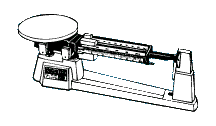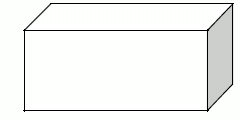# Metric Measurement Lab

 Metric Measurement LabTake a guess – How many drops of water will it take to equal 1 milliliter? _____ drops

Follow the directions to find the number of drops in 1 milliliter of water, then answer the questions. You will need a small graduated cylinder (25 ml), a beaker of water, and an eyedropper for this section.  Remember to read the bottom of the meniscus when you are reading the volume of a liquid in a graduated cylinder.

 Fill a small graduated cylinder with 10 ml of water.Count the number of drops it takes to raise the water to 11 ml. Record the number in the chart.Leave the water in the graduated cylinder and count the number of drops it takes to raise the water to 12ml. Record the number in the chart.Leave the water in the graduated cylinder and count the number of drops it takes to raise the water to 13ml. Record the number in the chart.Calculate your average and round to the nearest tenth.# of drops to 11 ml # of drops to 12 ml # of drops to 13 ml Average

Based on your average, how many drops would it take to make 1 liter? _______

Part B: Water Displacement

Follow the directions to find the volume of three marbles using water displacement.

1. Add 20 ml of water to a 100 ml graduated cylinder. Record this amount in the chart.
2. Add three marbles to the cylinder and measure the volume. Record this amount in the chart.
3. Find the difference between the two measurements and record in the chart. The difference between the two measurements will be the volume of the three marbles.
 Volume of Water Before adding Marbles (ml) Volume of Water After Adding Marbles (ml) Difference in Volume (ml) Volume of 3 Marbles

Part C: Mass Mania

The gram is the standard unit of mass in the metric or SI system. The basic instrument used to measure mass is the mass balance.  Some mass measurements can be made using an electronic balance.

 Check to see that the Pointer is pointing to zero.If it is not, check to see that all the Riders (weights) are all the way to the left at the Zero mark.Adjust the balance by turning the Adjustment Screw slowly until it points to zero.Place your metric ruler on the pan and read & record the ruler’s mass.After resetting the balance to Zero, measure and record the mass of the empty 50-ml graduated cylinder and then the 3 marbles.Reset the balance to ZERO when all items have been massed.Mass of Metric Ruler (g) Mass of Empty 50-ml graduated cylinder (g) Mass of 3 Marbles (g)

Part D: Volume by Formula

Use the formula to find the volume of the box. Measure to the nearest centimeter before calculating your answer.  If necessary, Round your answer to Two Decimal places.Volume = length x width x height

__________ x __________ x __________ =________________cm3

Part E: Color Challenge

1. Obtain the following items from your teacher:

• 3 beakers with colored water- 25 ml of each color (red, blue, and yellow)
• 1 graduated cylinder (25 ml – 50 ml)
• 1 eyedropper
• 6 test tubes labeled A, B, C, D, E, and F

2. Perform each step outlined below using accurate measurements.

1. Measure 17 ml of RED water from the beaker and pour into test tube A.
2. Measure 21 ml of YELLOW water from the beaker and pour into test tube C
3. Measure 22 ml of BLUE water from the beaker and pour into test tube E.
4. Measure 5 ml of water from test tube A and pour it into test tube B.
5. Measure 6 ml of water from test tube C and pour it into test tube D.
6. Measure 8 ml of water from test tube E and pour it into test tube F.
7. Measure 5 ml of water from test tube C and pour it into test tube B.
8. Measure 2 ml of water from test tube A and pour it into test tube F.
9. Measure 4 ml of water from test tube E and pour it into test tube D.

3. Complete the chart.

 Test Tube Color Final Volume (ml) A B C D E F